Tutorial index:

# 3. Neural networkAn artificial neural network, or simply a neural network, can be defined as a biologically inspired computational model that consists of a network architecture composed of artificial neurons. This structure contains a set of parameters, which can be adjusted to perform specific tasks.

Neural networks have universal approximation properties, which means that they can approximate any function in any dimension and up to a desired degree of accuracy.

The most used types of layers in approximationclassification and forecasting applications are the perceptron, probabilistic, long-short term memory (LSTM), scaling, unscaling, and bounding.

In other types of applications, such as computer vision or speech recognition, different layers, such as convolutional or associative, are commonly used.

## 3.1. Perceptron layer

The most critical layers of a neural network are the perceptron layers (also called dense layers). Indeed, they allow the neural network to learn.

The following figure shows a perceptron layer. It receives information as a set of numerical inputs. This information is then combined with the biases and the weights. Finally, the combinations are activated to produce the final outputs.The activation function determines the function that the layer represents. Some of the most common activation functions are the following:

### Linear activation function

The output of a perceptron with a linear activation function is simply the combination of that neuron.
$$activation = combination$$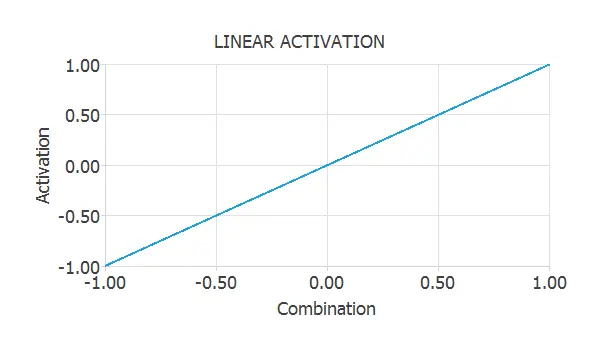### Hyperbolic tangent activation function

The hyperbolic tangent is one of the most used activation functions when constructing neural networks. It is a sigmoid function which varies between -1 and +1.
$$activation = tanh(combination)$$### Logistic activation function

The logistic is another type of sigmoid function. It is very similar to the hyperbolic tangent, but in this case, it varies between 0 and 1. $$activation = \frac{1}{1+e^{-combination}}$$### Rectified linear (ReLU) activation function

The rectified linear activation function, or ReLU, is one of the most used activation functions. It is zero when the combination is negative and equal to when the combination is zero or positive.
$$activation = \left\{ \begin{array}{lll} 0 &if& \textrm{combination < 0} \\ combination &if& \textrm{combination \geq 0} \end{array} \right.$$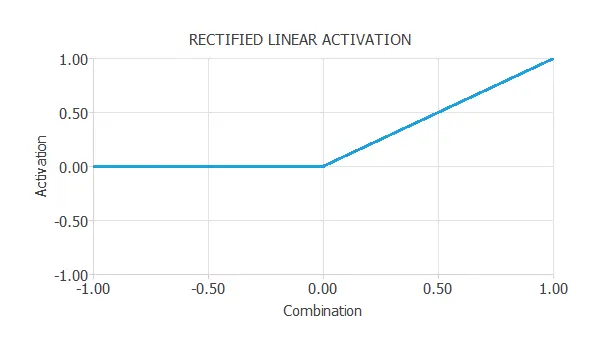You can read the article Perceptron: The main component of neural networks for a more detailed description of this critical neuron model.

In this regard, a perceptron layer is a group of neurons connecting to the same inputs and sending outputs to the same destinations.

### 3.2. Probabilistic layer

In classification problems, outputs are usually interpreted as class membership probabilities. In this way, the probabilistic outputs will fall in the range [0, 1], and the sum will be 1. The probabilistic layer provides outputs that can be interpreted as probabilities.

The following figure shows a probabilistic layer. It is very similar to the perceptron layer, but the activation functions are restricted to be probabilistic.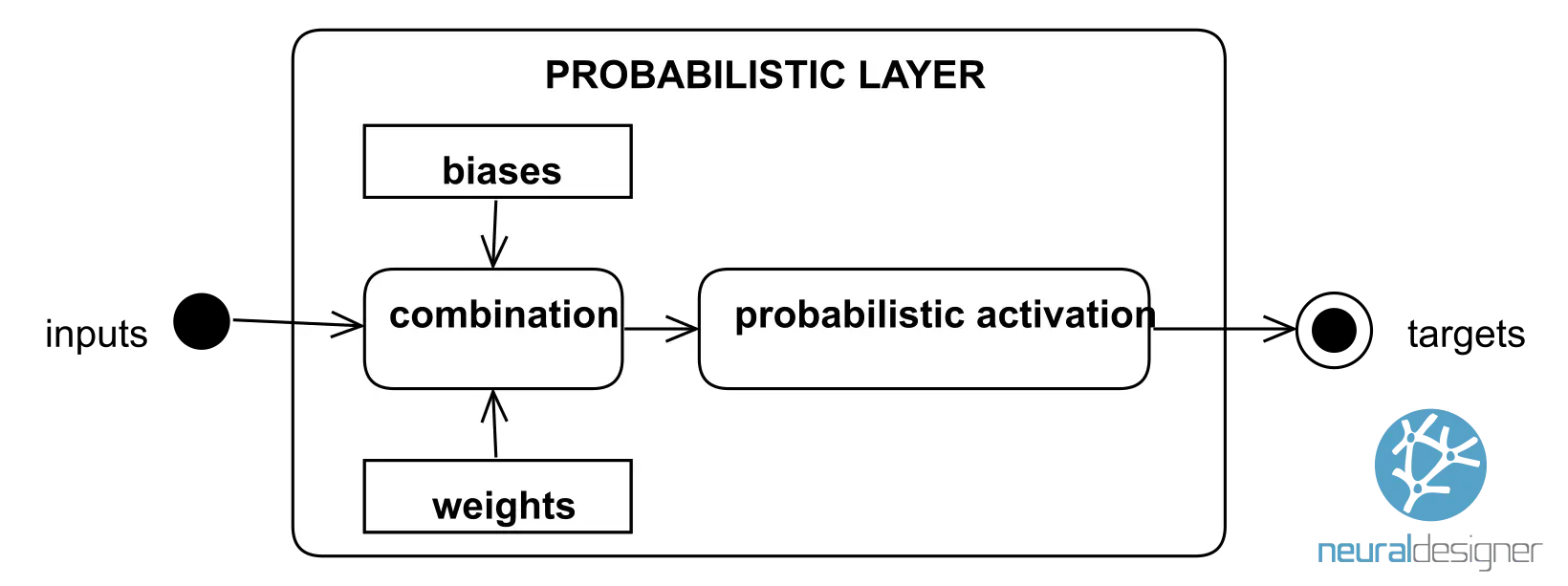Some of the most popular probabilistic activations are the following:

### Logistic probabilistic activation

The logistic activation function is used in binary classification applications. As we know, it is a sigmoid function that varies between 0 and 1.
$$probabilistic\_activation = \frac{1}{1+e^{-combination}}$$

### SoftMax probabilistic activation

This method is used in multiple classification problems. It is a continuous probabilistic function, which holds that the outputs always fall in the range [0, 1], and the sum of all is always 1.
$$probabilistic\_activation = \frac{e^{combination}}{\sum e^{combinations}}$$

## 3.3. Long-short term memory (LSTM) layer

Long-short term memory (LSTM) layers are a particular recurrent layer widely used in forecasting applications.

The following figure shows an LSTM layer. It receives information as a set of numerical inputs. This information is processed through forget, input, state, and output gates and stored in hidden and cell states. Finally, the layer produces the final outputs.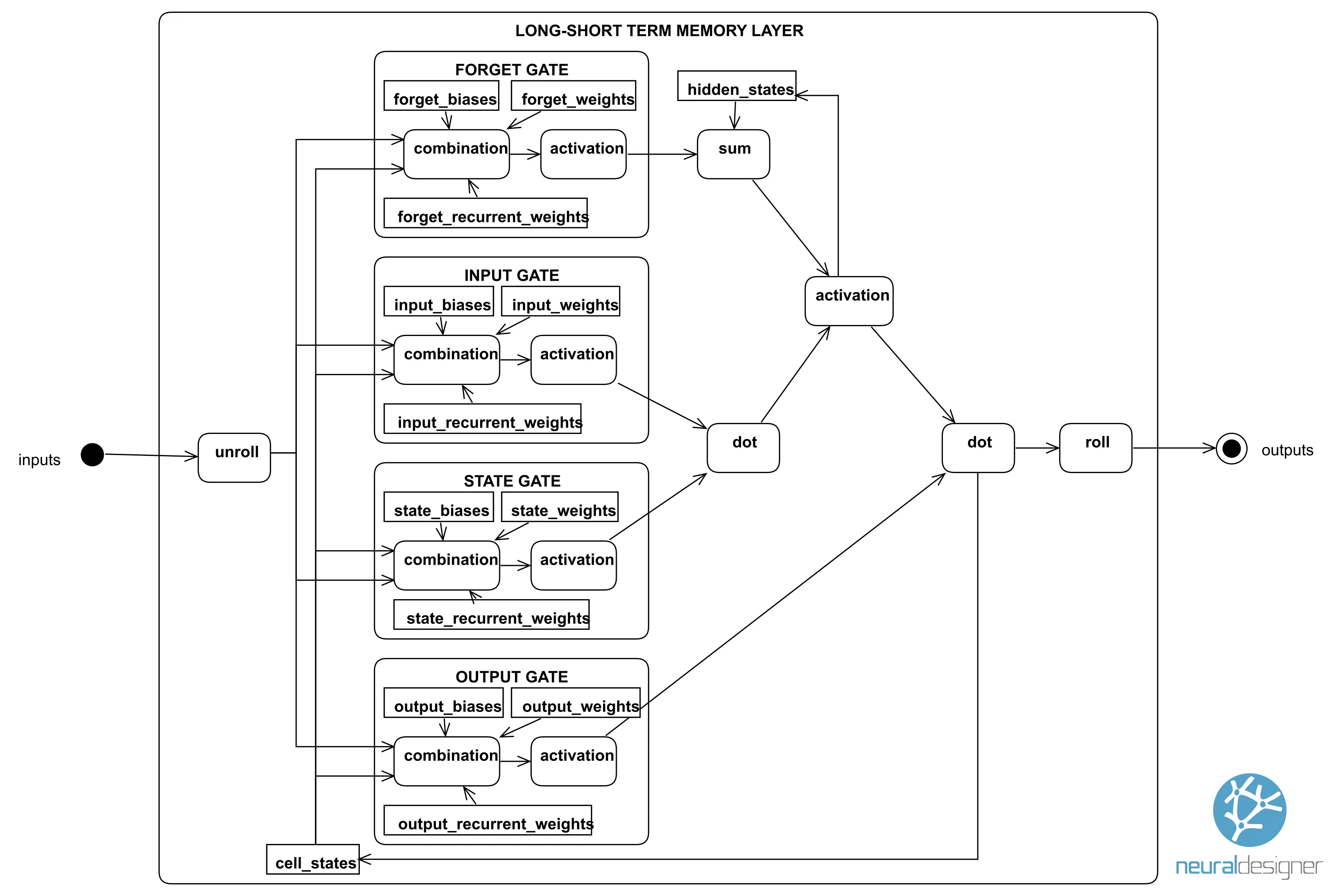As we can see, long-short term memory (LSTM) layers are complex and contain many parameters. That structure makes them suitable for learning dependencies from time-series data.

## 3.4. Scaling layer

In practice, it is always convenient to scale the inputs to give them a proper range. In the context of neural networks, the scaling layer performs this process.

The scaling layer contains some basic statistics on the inputs. They include the mean, standard deviation, minimum, and maximum values.

Some scaling methods used in practice are the following:

### Minimum and maximum scaling method

The minimum and maximum methods produce a data set scaled between −1 and 1. This method is usually applied to variables with a uniform distribution.
$$scaled\_input = -1+\frac{(input-minimum)\cdot (1-(-1))}{maximum-minimum}$$

### Mean and standard deviation scaling method

The mean and standard deviation method scales the inputs so that they will have mean 0 and standard deviation 1. This method usually applies to normal (or Gaussian) distribution variables.
$$scaled\_input = \frac{input-mean}{standard\_deviation}$$

### Standard deviation scaling method

The standard deviation scaling method produces inputs with standard deviation 1. This is usually applied to half-normal distributions,  variables centered at zero with only positive values.
$$scaled\_input = \frac{input}{standard\_deviation}$$

All scaling methods are linear and, in general, produce similar results. In all cases, the inputs’ scaling in the data set must be synchronized with the inputs’ scaling in the neural network. Neural Designer does that without any intervention by the user.

## 3.5. Unscaling layer

The scaled outputs from a neural network are unscaled to produce the original units. In the context of neural networks, the unscaling layer does this.

An unscaling layer contains some basic statistics on the outputs. They include the mean, standard deviation, minimum, and maximum values.

Four unscaling methods are used in practice:

### Minimum and maximum unscaling method

The minimum and maximum method unscales variables that have been previously scaled to have minimum -1 and maximum +1, to produce outputs in the original range,
$$unscaled\_output = minimum\\+scaled\_output\cdot(maximum-minimum)$$

### Mean and standard deviation unscaling method

The mean and standard deviation method unscales variables that have been previously scaled to have mean 0 and standard deviation 1,
$$unscaled\_output = mean\\+scaled\_output\cdot standard\_deviation$$

### Standard deviation unscaling method

The standard deviation method unscales variables that have been previously scaled to have a standard deviation of 1, to produce outputs in the original range,
$$unscaled\_output = scaled\_output\cdot standard\_deviation$$

### Logarithmic unscaling method

The logarithmic method unscales variables that have been previously subjected to a logarithmic transformation,
$$unscaled\_output = minimum\\+0.5(\exp{(scaled\_output)}+1)(maximum-minimum)$$

In all cases, the scaling of the targets in the data set must be synchronized with unscaling the outputs in the neural network. Neural Designer does that without any intervention by the user.

## 3.6. Bounding layer

The output needs to be limited between the two values in many cases. For instance, the quality of a product might be comprised of between 1 and 5 stars.

The bounding layer performs this task. It uses the following formula:

$$bounded\_output = \left\{ \begin{array}{l} lower\_bound, \quad \textrm{output < lower\_bound} \\ output, \quad \textrm{lower\_bound \leq output \leq upper\_bound} \\ upper\_bound, \quad \textrm{output \geq upper\_bound} \end{array} \right.$$

## 3.7. Network architecture

A neural network can be symbolized as a graph, where nodes represent neurons, and edges represent connectivities among neurons. An edge label represents the parameter of the neuron for which the flow goes in.

Most neural networks, even biological neural networks, exhibit a layered structure. Therefore, layers are the basis for determining the architecture of a neural network.

A neural network is built up by organizing layers of neurons in a network architecture. The characteristic network architecture here is the so-called feed-forward architecture. In a feed-forward neural network, layers are grouped into a sequence so that neurons in any layer are connected only to neurons in the next layer.

The following figure represents a neural network with four inputs, several layers of different types, and three outputs.## 3.8. Model parameters

The model parameters involve the parameters of each layer in the network architecture.

All these parameters can be grouped in a vector $\theta$, which can be written as
$$\theta = \left(\theta_1,\ldots,\theta_d \right).$$

The number of adaptable parameters, $d$, is the sum of parameters in each layer.

As we have seen, a neural network might be composed of different types of layers, depending on the needs of the predictive model.

Next, we describe each application type’s most common neural network configurations.

## 3.9. Approximation neural networks

An approximation the model usually contains a scaling layer, several perceptron layers, an unscaling layer, and a bounding layer.

Two layers of perceptrons will be enough to represent the data set most of the time. For very complex data sets, deeper architectures with three, four, or more layers of perceptrons might be required.

The following figure represents a neural network to estimate the power generated by a combined cycle power plant as a function of meteorological and plant variables.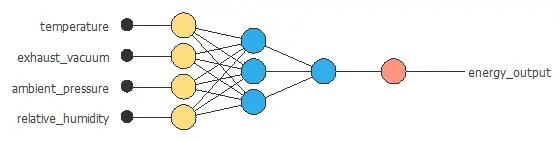The above neural network has four inputs and one output. It consists of a scaling layer (yellow), a perceptron layer with four neurons (blue), a perceptron layer with one neuron (blue), and an unscaling layer (red).

## 3.10. Classification of neural networks

classification the model usually requires a scaling layer, one or several perceptron layers, and a probabilistic layer. It might also contain a principal component layer.

Two layers of perceptrons will be enough to represent the data set most of the time.

The following figure is a binary classification model for diagnosing breast cancer from fine-needle aspirates.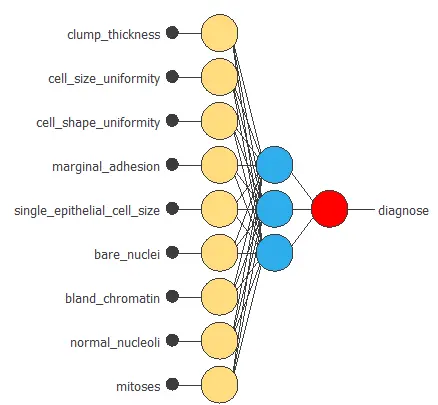The above neural network has nine inputs and one output. It consists of a scaling layer (yellow), a perceptron layer with three neurons (blue), a perceptron layer with one neuron (blue), and a probabilistic layer (red).

### 3.11. Forecasting neural networks

Forecasting applications are usually used for predicting a continuous variable. In this case, they might require a scaling layer, a long-short term memory layer, a perceptron layer, an unscaling layer and a bounding layer.

The following figure is a 1-day ahead forecasting model for the levels of NO2 in a city.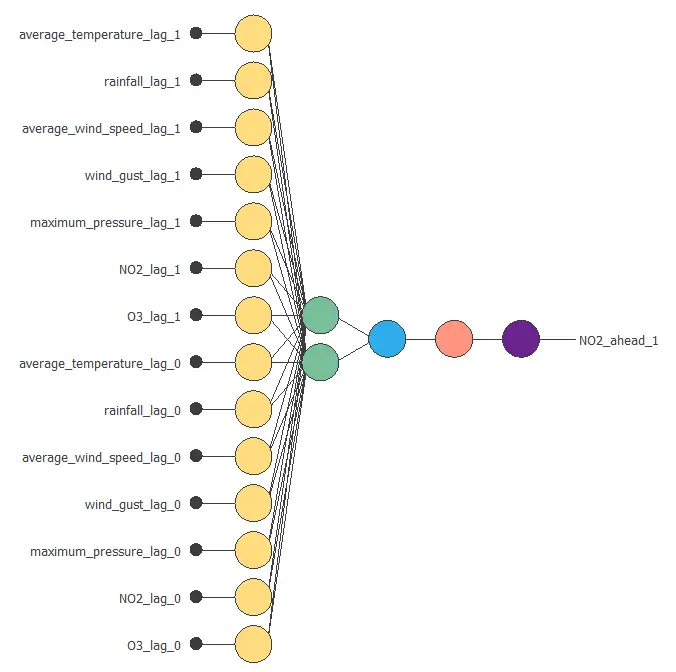The above neural network has 14 inputs and one output. It consists of a scaling layer (yellow), an LSTM layer with two neurons (green), a perceptron layer with one neuron (blue), an unscaling layer (red) and a bounding layer (blue).

In all cases, the inputs will contain lags variables, and the outputs will have steps ahead variables.